# Graph of an Equation

What is the Graph of an Equation?

The set of points where the equation is true.

### Example: y = x2 − 5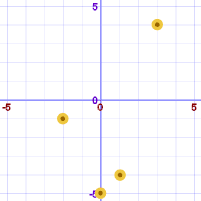We can plot some points where the equation is true:

x y = x2−5
−2 −1
0 −5
1 −4
3 4

Here we have a lot more points: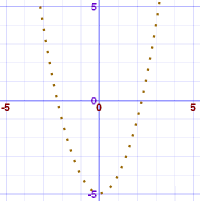And now the set of all points (within the range of the graph):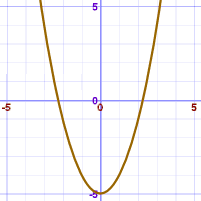Warning: Don't just plot a few points and think you have the graph!

### Example: y = x3 − 5x

With these calculated points:

x y = x3−5x
−2 2
0 0
2 −2

We might think this is the graph: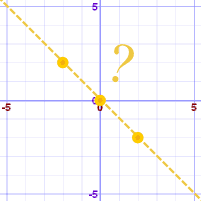But this is the real graph: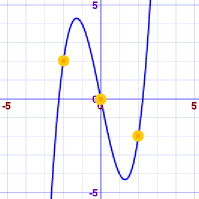## "Satisfy the Equation"

You may hear the phrase "satisfy the equation", which also means where the equation is true.

## How To Graph

• The Function Grapher can help you. Enter the equation as "y=(some function of x)". You can use zoom to find important points.
• If you can't write the equation as "y=(some function of x)", you can try the Equation Grapher, where you enter equations like "x^2+y^2=9" (meaning x2+y2=9).

But this is just a help! They are only computer programs, and could easily miss some important thing on the graph, or not plot something correctly.

In fact they are not really "Complete" Graphs unless they show all features ...

## A "Complete" Graph

For a graph to be "complete" we need to show all the important features.

• Peaks
• Valleys
• Flat areas
• Asymptotes
• Any other special features

Advanced Topic: Calculus (Finding Maxima and Minima using Derivatives) is a big help in finding important features.